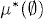# EXTENSION OF MEASURES

As defined in the previous chapter, a measure is a set function on an algebra. To extend a measure from an algebra to a σ-algebra, we need some tools. In this chapter, we will introduce concepts and theorems related to measure extension.

# 3.1 Basic Concepts and Facts

Definition 3.1 (Outer Measure). An outer measure on a setis a nonnegative, extended real-valued set functionthat satisfies the following conditions:

(a)= 0.
(b) (Monotonicity) If ABS, then μ*(A) ≤ μ* (B).
(c) (Countable subadditivity) If AnS for all n ≥ 1, thenDefinition 3.2 (Complete Measure). Let μ be a measure on a σ-algebra ∑. The measure μ, is said to be complete if and only if all subsets of a zero measure set in ∑ are in ∑. Thus, whenever A∑ with μ(A) = 0, we have B∑ for all BA.

Definition 3.3 (Completion of Measure Spaces). Let (S, ∑, μ) be a measure space. The completion ...

Get Measure, Probability, and Mathematical Finance: A Problem-Oriented Approach now with the O’Reilly learning platform.

O’Reilly members experience live online training, plus books, videos, and digital content from nearly 200 publishers.